Explanations supplement

This vignette provides additional documentation for some methods implemented in the emmeans package.

Index of all vignette topics

Sub-models

Estimated marginal means (EMMs) and other statistics computed by the emmeans package are model-based: they depend on the model that has been fitted to the data. In this section we discuss a provision whereby a different underlying model may be considered. The submodel option in update() can project EMMs and other statistics to an alternative universe where a simpler version of the model has been fitted to the data. Another way of looking at this is that it constrains certain external effects to be zero – as opposed to averaging over them as is otherwise done for marginal means.

Two things to know before getting into details:

1. The submodel option uses information from the fixed-effects portion of the model matrix
2. Not all model classes are supported for the submodel option.

Now some details. Suppose that we have a fixed-effects model matrix $$X$$, and let $$X_1$$ denote a sub-matrix of $$X$$ whose columns correspond to a specified sub-model. (Note: if there are weights, use $$X = W^{1/2}X^*$$, where $$X^*$$ is the model matrix without the weights incorporated.) The trick we use is what is called the alias matrix: $$A = (X_1'X_1)^-X_1'X$$ where $$Z^-$$ denotes a generalized inverse of $$Z$$. It can be shown that $$(X_1'X_1)^-X_1' = A(X'X)^-X'$$; thus, in an ordinary fixed-effects regression model, $$b_1 = Ab$$ where $$b_1$$ and $$b$$ denote the regression coefficients for the sub-model and full model, respectively. Thus, given a matrix $$L$$ such that $$Lb$$ provides estimates of interest for the full model, the corresponding estimates for the sub-model are $$L_1b_1$$, where $$L_1$$ is the sub-matrix of $$L$$ consisting of the columns corresponding to the columns of $$X_1$$. Moreover, $$L_1b_1 = L_1(Ab) = (L_1A)b$$; that is, we can replace $$L$$ by $$L_1A$$ to obtain estimates from the sub-model. That’s all that update(..., submodel = ...) does.

Here are some intuitive observations:

1. Consider the excluded effects, $$X_2$$, consisting of the columns of $$X$$ other than $$X_1$$. The corresponding columns of the alias matrix are regression coefficients treating $$X_2$$ as the response and $$X_1$$ as the predictors.
2. Thus, when we obtain predictions via these aliases, we are predicting the effects of $$X_2$$ based on $$X_1$$.
3. The columns of the new linear predictor $$\tilde L = L_1A$$ depend only on the columns of $$L_1$$, and hence not on other columns of $$L$$.

These three points provide three ways of saying nearly the same thing, namely that we are excluding the effects in $$X_2$$. Note that in a rank-deficient situation, there are different possible generalized inverses, and so in (1), $$A$$ is not unique. However, the predictions in (2) are unique. In ordinary regression models, (1), (2), and (3) all apply and will be the same as predictions from re-fitting the model with model matrix $$X_1$$; however, in generalized linear models, mixed models, etc., re-fitting will likely produce somewhat different results. That is because fitting such models involves iterative weighting, and the re-fitted models will probably not have the same weights. However, point (3) will still hold: the predictions obtained with a submodel will involve only the columns of $$L_1$$ and hence constrain all effects outside of the sub-model to be zero. Therefore, when it really matters to get the correct estimates from the stated sub-model, the user should actually fit that sub-model unless the full model is an ordinary linear regression.

A technicality: Most writers define the alias matrix as $$(X_1'X_1)^-X_1'X_2$$, where $$X_2$$ denotes that part of $$X$$ that excludes the columns of $$X_1$$. We are including all columns of $$X$$ here just because it makes the notation very simple; the $$X_1$$ portion of $$X$$ just reduces to the identity (at least in the case where $$X_1$$ is full-rank).

A word on computation: Like many matrix expressions, we do not compute $$A$$ directly as shown. Instead, we use the QR decomposition of $$X_1$$, obtainable via the R call Z <- qr(X1). Then the alias matrix is computed via A <- qr.coef(Z, X). In fact, nothing changes if we use just the $$R$$ portion of $$X = QR$$, saving us both memory and computational effort. The exported function .cmpMM() extracts this $$R$$ matrix, taking care of any pivoting that might have occurred. And in an lm object, the QR decomposition of $$X$$ is already saved as a slot. The qr.coef() function works just fine in both the full-rank and rank-deficient cases, but in the latter situation, some elements of A will be NA; those correspond to “excluded” predictors, but that is another way of saying that we are constraining their regression coefficients to be zero. Thus, we can easily clean that up via A[is.na(A)] <- 0.

If we specify submodel = "minimal", the software figures out the sub-model by extracting terms involving only factors that have not already been averaged over. If the user specifies submodel = "type2", an additional step is performed: Let $$X_1$$ have only the highest-order effect in the minimal model, and $$X_0$$ denote the matrix of all columns of $$X$$ whose columns do not contain the effect in $$X_1$$. We then replace $$Z$$ by the QR decomposition of $$[I - X_0(X_0'X_0)^-X_0']X_1^*$$. This projects $$X_1^*$$ onto the null space of $$X_0$$. The net result is that we obtain estimates of just the $$X_1^*$$ effects, after adjusting for all effects that don’t contain it (including the intercept if present). Such estimates have very limited use in data description, but provide a kind of “Type II” analysis when used in conjunction with joint_tests(). The "type2" calculations parallel those documented by SAS for obtaining type II estimable functions in SAS PROC GLM. However, we (as well as car::Anova()) define “contained” effects differently from SAS, treating covariates no differently than factors.

A note on multivariate models

Recall that emmeans generates a constructed factor for the levels of a multivariate response. That factor (or factors) is completely ignored in any sub-model calculations. The $$X$$ and $$X_1$$ matrices described above involve only the predictors in the right-hand side of the model equation . The multivariate response “factor” implicitly interacts with everything in the right-hand-side model; and the same is true of any sub-model. So it is not possible to consider sub-models where terms are omitted from among those multivariate interactions (note that it is also impossible to fit a multivariate sub-model that excludes those interactions). The only way to remove consideration of multivariate effects is to average over them via a call to emmeans().

Back to Contents

Comparison arrows

The plot() method for emmGrid objects offers the option comparisons = TRUE. If used, the software attempts to construct “comparison arrows” whereby two estimated marginal means (EMMs) differ significantly if, and only if, their respective comparison arrows do not overlap. In this section, we explain how these arrows are obtained.

First, please understand these comparison arrows are decidedly not the same as confidence intervals. Confidence intervals for EMMs are based on the statistical properties of the individual EMMs, whereas comparison arrows are based on the statistical properties of differences of EMMs.

Let the EMMs be denoted $$m_1, m_2, ..., m_k$$. For simplicity, let us assume that these are ordered: $$m_1 \le m_2 \le \cdots \le m_k$$. Let $$d_{ij} = m_j - m_i$$ denote the difference between the $$i$$th and $$j$$th EMM. Then the $$(1 - \alpha)$$ confidence interval for the true difference $$\delta_{ij} = \mu_j - \mu_i$$ is $d_{ij} - e_{ij}\quad\mbox{to}\quad d_{ij} + e_{ij}$ where $$e_{ij}$$ is the “margin of error” for the difference; i.e., $$e_{ij} = t\cdot SE(d_{ij})$$ for some critical value $$t$$ (equal to $$t_{\alpha/2}$$ when no multiplicity adjustment is used). Note that $$d_{ij}$$ is statistically significant if, and only if, $$d_{ij} > e_{ij}$$.

Now, how to get the comparison arrows? These arrows are plotted with origins at the $$m_i$$; we have an arrow of length $$L_i$$ pointing to the left, and an arrow of length $$R_i$$ pointing to the right. To compare EMMs $$m_i$$ and $$m_j$$ (and remembering that we are supposing that $$m_i \le m_j$$), we propose to look to see if the arrows extending right from $$m_i$$ and left from $$m_j$$ overlap or not. So, ideally, if we want overlap to be identified with statistical non-significance, we want $R_i + L_j = e_{ij} \quad\mbox{for all } i < j$

If we can do that, then the two arrows will overlap if, and only if, $$d_{ij} < e_{ij}$$.

This is easy to accomplish if all the $$e_{ij}$$ are equal: just set all $$L_i = R_j = \frac12e_{12}$$. But with differing $$e_{ij}$$ values, it may or may not even be possible to obtain suitable arrow lengths.

The code in emmeans uses an ad hoc weighted regression method to solve the above equations. We give greater weights to cases where $$d_{ij}$$ is close to $$e_{ij}$$, because those are the cases where it is more critical that we get the lengths of the arrows right. Once the regression equations are solved, we test to make sure that $$R_i + L_j < d_{ij}$$ when the difference is significant, and $$\ge d_{ij}$$ when it is not. If one or more of those checks fails, a warning is issued.

That’s the essence of the algorithm. Note, however, that there are a few complications that need to be handled:

• For the lowest EMM $$m_1$$, $$L_1$$ is completely arbitrary because there are no right-pointing arrows with which to compare it; in fact, we don’t even need to display that arrow. The same is true of $$R_k$$ for the largest EMM $$m_k$$. Moreover, there could be additional unneeded arrows when other $$m_i$$ are equal to $$m_1$$ or $$m_k$$.
• Depending on the number $$k$$ of EMMs and the number of tied minima and maxima, the system of equations could be under-determined, over-determined, or just right.
• It is possible that the solution could result in some $$L_i$$ or $$R_j$$ being negative. That would result in an error.

In summary, the algorithm does not always work (in fact it is possible to construct cases where no solution is possible). But we try to do the best we can. The main reason for trying to do this is to encourage people to not ever use confidence intervals for the $$m_i$$ as a means of testing the comparisons $$d_{ij}$$. That is almost always incorrect.

What is better yet is to simply avoid using comparison arrows altogether and use pwpp() or pwpm() to display the P values directly.

Examples and tests

Here is a constructed example with specified means and somewhat unequal SEs

m = c(6.1, 4.5, 5.4,    6.3, 5.5, 6.7)
se2 = c(.3, .4, .37,  .41, .23, .48)^2
lev = list(A = c("a1","a2","a3"), B = c("b1", "b2"))
foo = emmobj(m, diag(se2), levels = lev, linfct = diag(6))
plot(foo, CIs = FALSE, comparisons = TRUE)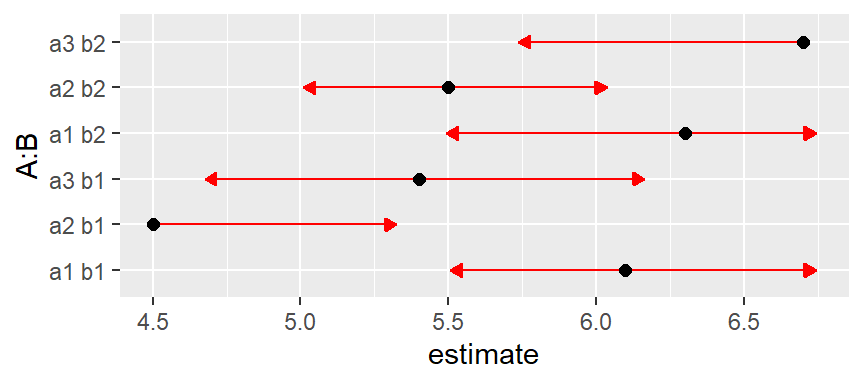This came out pretty well. But now let’s keep the means and SEs the same but make them correlated. Such correlations happen, for example, in designs with subject effects. The function below is used to set a specified intra-class correlation, treating A as a within-subjects (or split-plot) factor and B as a between-subjects (whole-plot) factor. We’ll start with a correlation of 0.3.

mkmat <- function(V, rho = 0, indexes = list(1:3, 4:6)) {
sd = sqrt(diag(V))
for (i in indexes)
V[i,i] = (1 - rho)*diag(sd[i]^2) + rho*outer(sd[i], sd[i])
V
}
# Intraclass correlation = 0.3
foo3 = foo
foo3@V <- mkmat(foo3@V, 0.3)
plot(foo3, CIs = FALSE, comparisons = TRUE)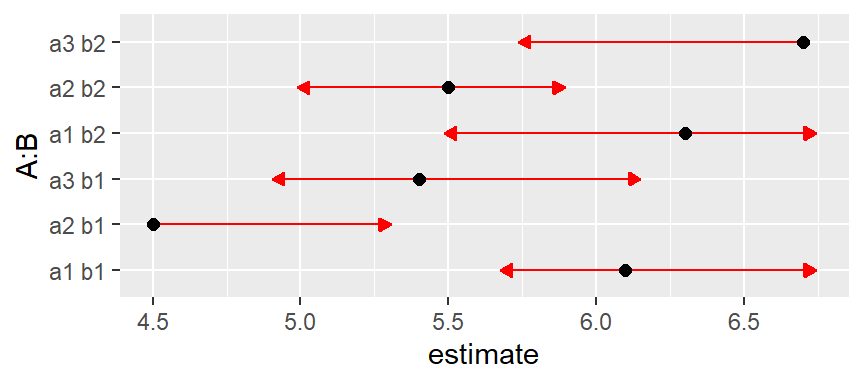Same with intraclass correlation of 0.6:

foo6 = foo
foo6@V <- mkmat(foo6@V, 0.6)
plot(foo6, CIs = FALSE, comparisons = TRUE)
## Warning: Comparison discrepancy in group "1", a1 b1 - a2 b2:
##     Target overlap = 0.443, overlap on graph = -0.2131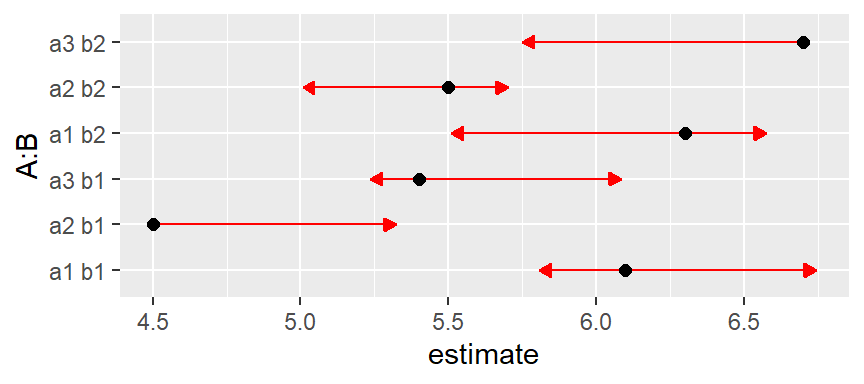Now we have a warning that some arrows don’t overlap, but should. We can make it even worse by upping the correlation to 0.8:

foo8 = foo
foo8@V <- mkmat(foo8@V, 0.8)
plot(foo8, CIs = FALSE, comparisons = TRUE)
## Error: Aborted -- Some comparison arrows have negative length!
## (in group "1")

Now the solution actually leads to negative arrow lengths.

What is happening here is we are continually reducing the SE of within-B comparisons while keeping the others the same. These all work out if we use B as a by variable:

plot(foo8, CIs = FALSE, comparisons = TRUE, by = "B")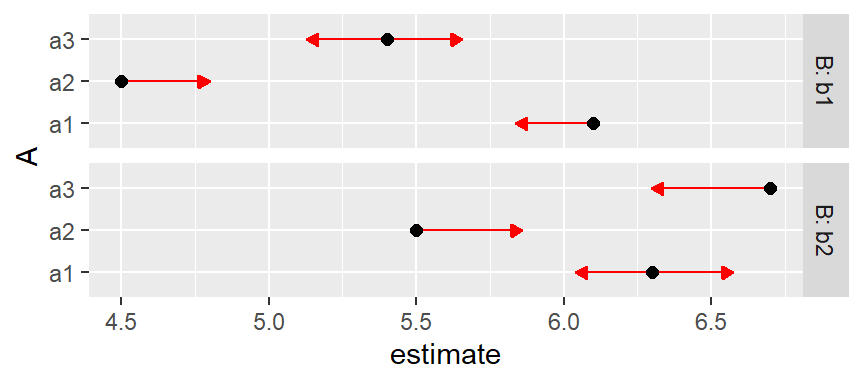Note that the lengths of the comparison arrows are relatively equal within the levels of B. Or, we can use pwpp() or pwpm() to show the P values for all comparisons among the six means:

pwpp(foo6, sort = FALSE)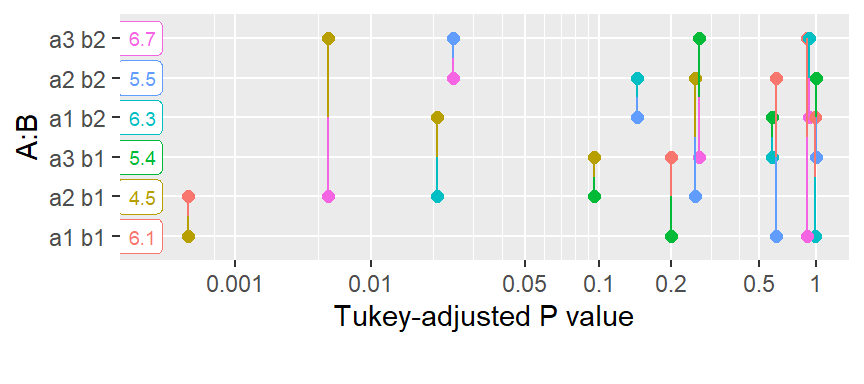pwpm(foo6)
##       a1 b1  a2 b1  a3 b1  a1 b2  a2 b2  a3 b2
## a1 b1 [6.1] <.0001 0.1993 0.9988 0.6070 0.8972
## a2 b1   1.6  [4.5] 0.0958 0.0208 0.2532 0.0057
## a3 b1   0.7   -0.9  [5.4] 0.5788 0.9999 0.2641
## a1 b2  -0.2   -1.8   -0.9  [6.3] 0.1439 0.9204
## a2 b2   0.6   -1.0   -0.1    0.8  [5.5] 0.0245
## a3 b2  -0.6   -2.2   -1.3   -0.4   -1.2  [6.7]
##
## Row and column labels: A:B
## Upper triangle: P values   adjust = "tukey"
## Diagonal: [Estimates] (estimate)
## Lower triangle: Comparisons (estimate)   earlier vs. later

Back to Contents

Index of all vignette topics